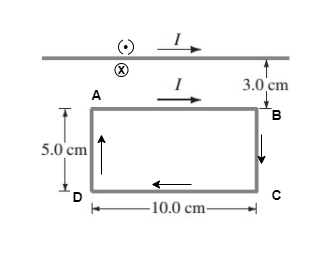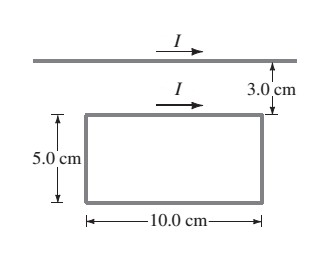# Problem: A rectangular loop of wire is placed next to a straight wire, as shown in the (Figure 1) . There is a current of I = 6.5A in both wires. A. Determine the direction of the net force on the loop. B. Determine the magnitude of the net force on the loop.

###### FREE Expert Solution

Magnetic force on the loop:

$\overline{){\mathbf{F}}{\mathbf{=}}\frac{{\mathbf{\mu }}_{\mathbf{0}}\mathbf{li}}{\mathbf{2}\mathbf{\pi }\mathbf{r}}}$

Let's label the diagram as:A.

AD and BC are symmetrically placed with respect to the straight wire and carry opposite currents.

Therefore, the resultant force on AD and BC is zero.

AB is nearer to the straight wire than DC thereby experiencing more force.

99% (40 ratings)###### Problem Details

A rectangular loop of wire is placed next to a straight wire, as shown in the (Figure 1) . There is a current of I = 6.5A in both wires.A. Determine the direction of the net force on the loop.

B. Determine the magnitude of the net force on the loop.